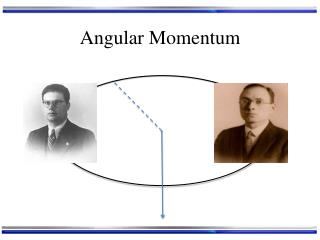DownloadDownload PresentationAngular Momentum

# Angular Momentum

Télécharger la présentation## Angular Momentum

- - - - - - - - - - - - - - - - - - - - - - - - - - - E N D - - - - - - - - - - - - - - - - - - - - - - - - - - -
##### Presentation Transcript

1. Angular Momentum

2. What was Angular Momentum Again? • If a particle is confined to going around a sphere: At any instant the particle is on a particular circle r The particle is some distance from the origin, r The particle has angular momentum, L = r × p The particle or mass m has some velocity, v and momentum p v

3. What was Angular Momentum Again? • So a particle going around in a circle (at any instant) has angular momentum L: L = r × p Determine L’s direction from the “right hand rule” p r

4. What was Angular Momentum Again? • Llike any 3D vector has 3 components: • Lx : projection of L on a x-axis • Ly: projection of L on a y-axis • Lz : projection of L on a z-axis L = r × p z p x r y

5. What was Angular Momentum Again? • Picking up L and moving it over to the origin: L = r × p L = r × p z p x r y

6. What was Angular Momentum Again? • Picking up L and moving it over to the origin: L = r × p z Rotate x y

7. What was Angular Momentum Again? • And re-orienting: Rotate L = r × p z x y

8. What was Angular Momentum Again? • And re-orienting: L = r × p z x y • Now we’re in a viewpoint that will be convenient to analyse

9. Angular Momentum Operator • L is important to us because electrons are constantly changing direction (turning) when they are confined to atoms and molecules • L is a vector operator in quantum mechanics • Lx: operator for projection of L on a x-axis • Ly: operator for projection of L on a y-axis • Lz : operator for projection of L on a z-axis

10. Angular Momentum Operator • Just for concreteness L is written in terms of position and momentum operators as: with

11. Angular Momentum Operators • Ideally we’d like to know L BUT… • Lx , Lyand Lz don’t commute! • By Heisenberg, we can’t measure them simultaneously, so we can’t know exactly where and what L is! One day this will be a lab…

12. Angular Momentum Operators • does commute with each of • , and individually • is the length of L squared. • has the simplest mathematical form • So let’s pick the z-axis as our “reference” axis

13. Angular Momentum Operators • So we’ve decided that we will use and as a substitute for • Because we can simultaneously measure: • L2 the length of L squared • Lz the projection of L on the z-axis z BUT we can’t know Lx, Ly and Lz simultaneously! We’ve chosen to know only Lz (and L2) x L y Lz Ly Lx

14. Angular Momentum Operators • So we’ve decided that we will use and as a substitute for • Because we can simultaneously measure: • L2 the length of L squared • Lz the projection of L on the z-axis z x For different L2’s we’ll have different Lz’s L y Lz So what are the possible and eigenvalues and what are their eigen-functions? can be anywhere in a cone for a given Lz

15. Angular Momentum Eigen-System • Operators that commute have the same eigenfunctions • and commute so they have the same eigenfunctions • Using the commutation relations on the previous slides along with: • we’d find…. One day this will be a lab too…

16. Angular Momentum Eigen-System • EigenfunctionsY, called: Spherical Harmonics • l = {0,1,2,3,….} angular momentum quantum number • ml = {-l, …, 0, …, l} magnetic quantum number

17. Angular Momentum Vector Diagrams z Say l = 2 then m ={-2, -1, 0, 1, 2} For m =2 For m =2

18. Angular Momentum Vector Diagrams z • Take home messages: • The magnitude (length) of angular momentum is quantized: • Angular momentum can only point in certain directions: • Dictated by l and m

19. Angular Momentum Eigenfunctions • The explicit form of and is best expressed in spherical polar coordinates: z For now, our particle is on a sphere and r is constant q r y f x • We won’t actually formulate these operators (they are too messy!), but their wave functions Y, will be in terms of q and f instead of x, y and z: • Yl,m(q,f) = Ql,m(q) Fm(f)

20. Angular Momentum Eigenfunctions • l = 0, ml= 0

21. Angular Momentum Eigenfunctions l = 1 ml={-1, 0, 1} Look Familiar?

22. Angular Momentum Eigenfunctions l = 2 ml={-2, -1, 0, 1, 2} Look Familiar?

23. Particle on a Sphere r qcan vary form 0 to p fcan vary form 0 to2 p r is constant

24. Particle on a Sphere • The Schrodinger equation: 0

25. Particle on a Sphere • So for particle on a sphere: Legendre Polynomials Spherical harmonics • Energies are 2l + 1 fold degenerate since: • For each l, there are {ml} = 2l + 1 eigenfunctions of the same energy